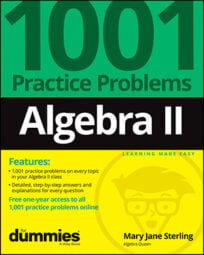##### Algebra II: 1001 Practice Problems For Dummies (+ Free Online Practice)The best way to figure out how the different algebraic rules work and interact with one another is to practice with lots of problems. And Algebra II requires lots of practice. So be prepared to solve equations and systems, graph lines, tackle functions, and so much more.

## Working with radical and rational equations

A radical equation is one that starts out with a square root, cube root, or some other root and gets changed into another form to make the solving process easier. A rational equation is one that involves a fractional expression — usually with a polynomial in the numerator and denominator. Avoid these mistakes when working with radical or rational equations:

• Forgetting to check for extraneous solutions

• Squaring a binomial incorrectly when squaring both sides to get rid of the radical

• Distributing correctly when writing equivalent fractions using a common denominator

• Eliminating solutions that create a 0 in the denominator

## Polynomial functions and equations

In Algebra II, a polynomial function is one in which the coefficients are all real numbers, and the exponents on the variables are all whole numbers. A polynomial whose greatest power is 2 is called a quadratic polynomial; if the highest power is 3, then it’s called a cubic polynomial. A highest power of 4 earns the name quartic (not to be confused with quadratic), and a highest power of 5 is called quintic.

When solving polynomial functions and equations, don’t let these common mistakes trip you up:

• Forgetting to change the signs in the factored form when identifying x-intercepts

• Making errors when simplifying the terms in f(–x) applying Descartes’ rule of sign

• Not changing the sign of the divisor when using synthetic division

• Not distinguishing between curves that cross from those that just touch the x-axis at an intercept

• Graphing the incorrect end-behavior on the right and left of the graphs

## Systems of linear equations

In Algebra II, a linear equation consists of variable terms whose exponents are always the number 1. When you have two variables, the equation can be represented by a line. With three terms, you can draw a plane to describe the equation. More than three variables is indescribable, because there are only three dimensions. When you have a system of linear equations, you can find the values of the variables that work for all the equations in the system — the common solutions. Sometimes there’s just one solution, sometimes many, and sometimes there’s no solution at all.

When solving systems of linear equations, watch out for these mistakes:

• Forgetting to change the signs in the factored form when identifying x-intercepts

• Making errors when simplifying the terms in f(–x) applying Descartes’ rule of sign

• Not changing the sign of the divisor when using synthetic division

• Not distinguishing between curves that cross from those that just touch the x-axis at an intercept

• Graphing the incorrect end-behavior on the right and left of the graphs